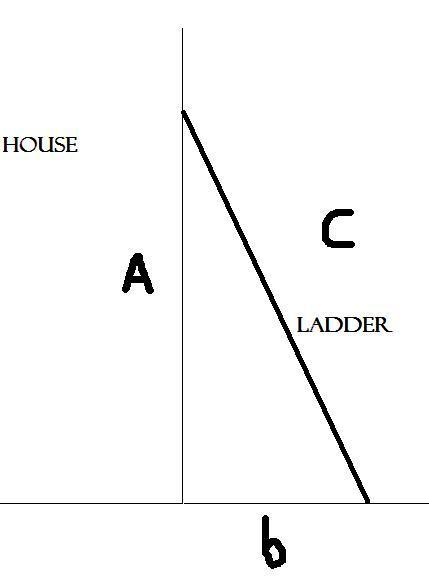• PEZenfuego

#### PEZenfuego

1. Homework Statement [/b]
This is a pretty simple calculus problem, but I can't figure out why my method fails.

A ladder 20 feet long is leaning against the wall of a house. The base of the ladder is pulled away from the wall at a rate of 4 ft/s. Consider the triangle formed by the side of the house, the ladder, and the ground. Find the rate at which the area of the triangle is changed when the base of the ladder is 9 feet from the wall.a=?
b=9 ft
c=20 ft
A=?
da/dt=?
db/dt=4 ft/s
dA/dt=?

c=a^2+b^2
A=(1/2)ab

## The Attempt at a Solution

Well, I am less interested in the actual solution and more interested in why my attempt at the solution did not work.

I began by realizing that the rate of change of c would be 0, since the ladder will not become longer or shorter. Then I did this:
c^2=a^2 + b^2
c^2-b^2=a^2
a=(c^2-b^2)^1/2

A=(1/2)ba
A=(1/2)b(c^2-b^2)^1/2
I am deriving with respect to b
dA/db=(1/2)((db/db)(c^2-b^2)^1/2+(b(1/2(c^2-b^2)^-1/2)(2c(dc/db)-2b(db/db)))))
and then simplify where (dc/db=0) and where (db/db=1)
dA/db=(1/2)((c^2-b^2)^1/2+(1/2(b(c^2-b^2)^-1/2)(-2b)))

And

(dA/db)x(db/dt)=dA/dt so all that I have to do is multiply the answer I just found by the rate at db/dt which I already know. The answer I find is 26.65 which I have been told is wrong. Where did I go wrong and how do I go about solving this problem?

Last edited:

## Homework Statement

This is a pretty simple calculus problem, but I can't figure out why my method fails.

A ladder 20 feet long is leaning against the wall of a house. The base of the ladder is pulled away from the wall at a rate of 4 ft/s. Consider the triangle formed by the side of the house, the ladder, and the ground. Find the rate at which the area of the triangle is changed when the base of the ladder is 9 feet from the wall.

a=?
b=9 ft
c=20 ft
A=?
da/dt=?
db/dt=4 ft/s
dA/dt=?

c=a^2+b^2
A=(1/2)ab

## The Attempt at a Solution

Well, I am less interested in the actual solution and more interested in why my attempt at the solution did not work.

I began by realizing that the rate of change of c would be 0, since the ladder will not become longer or shorter. Then I did this:
c^2=a^2 + b^2
c^2-b^2=a^2
a=(c^2-b^2)^1/2

A=(1/2)ba
A=(1/2)b(c^2-b^2)^1/2
I am deriving with respect to b
dA/db=(1/2)((db/db)(c^2-b^2)^1/2+(b(1/2(c^2-b^2)^-1/2)(2c(dc/db)-2b(db/db)))))
and then simplify where (dc/db=0) and where (db/db=1)
dA/db=(1/2)((c^2-b^2)^1/2+(1/2(b(c^2-b^2)^-1/2)(-2b)))

And

(dA/db)x(db/dt)=dA/dt so all that I have to do is multiply the answer I just found by the rate at db/dt which I already know. The answer I find is 26.65 which I have been told is wrong. Where did I go wrong and how do I go about solving this problem?

I get the same answer you do, so I don't see what you are doing wrong.